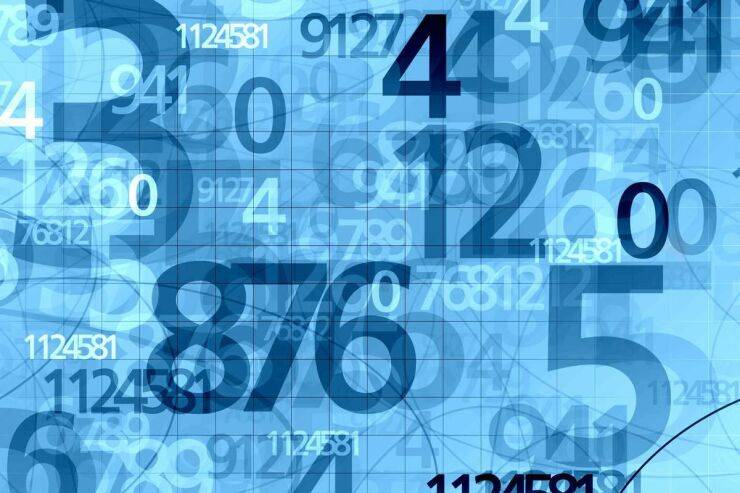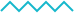Slide 1
What's in a Number?...Thus in Geometry, a number represents a Length or a Ratio or a Quantity. In Arithmetic, it is always an identity; in Calculus, the limit of a function. In mathematical and philosophic theory, every number is infinite in itself...

## What is a Number?

There is a philosophy of mathematics and a Science on the theory of Numbers that has discussed this question and de-constructed our notions of Number, and lain bare the deficiencies of our Understanding on this matter better than we can hope to do so here. We remind the Student however, that in Mathematics itself, even a thing so simple as Number is defined according to its scope and needs. Thus in Geometry, a number represents a Length or a Ratio or a Quantity. In Arithmetic, it is always an identity; in Calculus, the limit of a function. In mathematical and philosophic theory, every number is infinite in itself and contains within it the possibility for engendering all other Identities by combining with other Numbers. Even the transcendental numbers which cannot be expressed finitely, can be written down as the operation between two numbers to some degree.1 Thus ∏, though incomprehensible as a string of numbers, is simply the circumference of the Circle divided by its diameter and expressible as a simple ratio of two quantities. Though the result is mathematically, a fiction; we allow it into our calculations, for we witness Circles in Nature and know this ratio to exist in Reality, though it does not in our current Field of integral Number.2

## Integer Properties (N=1 to 2000)

THE LEXICON appended to this work tabulates the integers along with their list of factors and properties if any. Among these properties we include:

1. Prime or composite (Prime numbers indicated; otherwise the integer is composite.)1
2. Whether the number be the termination of a series (e.g. 276 = ∑23)
3. Whether the integer is a perfect number or not.
4. A perfect square.
5. Any other special properties.

## Significance of the Integers

 Numeral Mystical Interpretation Derivation 0 The Void, The Yoni, the Egg, the receptacle, the all-absorbing, no reflection. 1 Consciousness, The Phallus, reproducing after itself, the all-reflecting. From 0 by extension 2 Consciousness aware of itself through reflection, thought, sound, reverberation. From 1 by reflection 1/1 (or by revolution of the line around its end) 3 Consciousness within and a part of Nuit, aware of itself, all-relation. From 1 and 2 by addition 4 Consciousness manifest (and profane), aware of itself as independent to all relation. From 2 by multiplication. 5 Consciousness aware and active upon the field of relation. From 2 and 3 by addition. 6 Consciousness in perfect relation, passive and balanced. From 2 and 3 by multiplication. 7 Consciousness unites through a particular relation. Union. From 2 and 3 by multiplication. 8 Consciousness perceives itself materially in this relation. From 3 and 4 by addition, also 8 = 23. 9 Consciousness wills this relation. From 2 and 3 by multiplication, also 9 = 32. 10 Consciousness (1) separate from Potential (0) for the possibility of Union. From 1 + 2 + 3 + 4.

## The Decimal System

 0 1 2 3 4 5 6 7 8 9

THE Arabic numeral set and the decimal numerical system is the accepted standard on the planet today by which one can affirm quantity or classify a given identity. It is called the decimal system because it consists of ten elements (0 to 9) arranged in increasing powers of 10n, n ϵ I from left to right. Thus 3213.56 is no more than 3 × 103 + 2 × 102 + 1 × 101 + 3 × 100 + 5 × 10-1 + 3 × 10-2. However, the decimal system was not the first of the numerical systems employed nor by any means the more practical or best-suited. There is some belief that the decimal systems arises from the fact that human beings have ten fingers. History presents evidence that suggests the decimal system was introduced as a very deliberate and enforced standard that did not arise naturally and gain preponderance throughout the world through the process of natural selection as some historians have argued.

## Definitions

A set is a collection of things. In mathematics, we deal primarily with sets of numbers; in Qabalah with letters or words. The most common sets in mathematics are the sets natural numbers and the integers.

In this case, we will be looking at sets of letters (the English Alphabet) and sets of Natural Numbers (1,2, 3,… etc). We define them as follows:

• Let Q be the set of English Letters, that is Q = {A, B, C,…, Z}.
• Let N be the set of Natural Numbers. N = {1, 2, 3, 4, ... } = the natural numbers3.